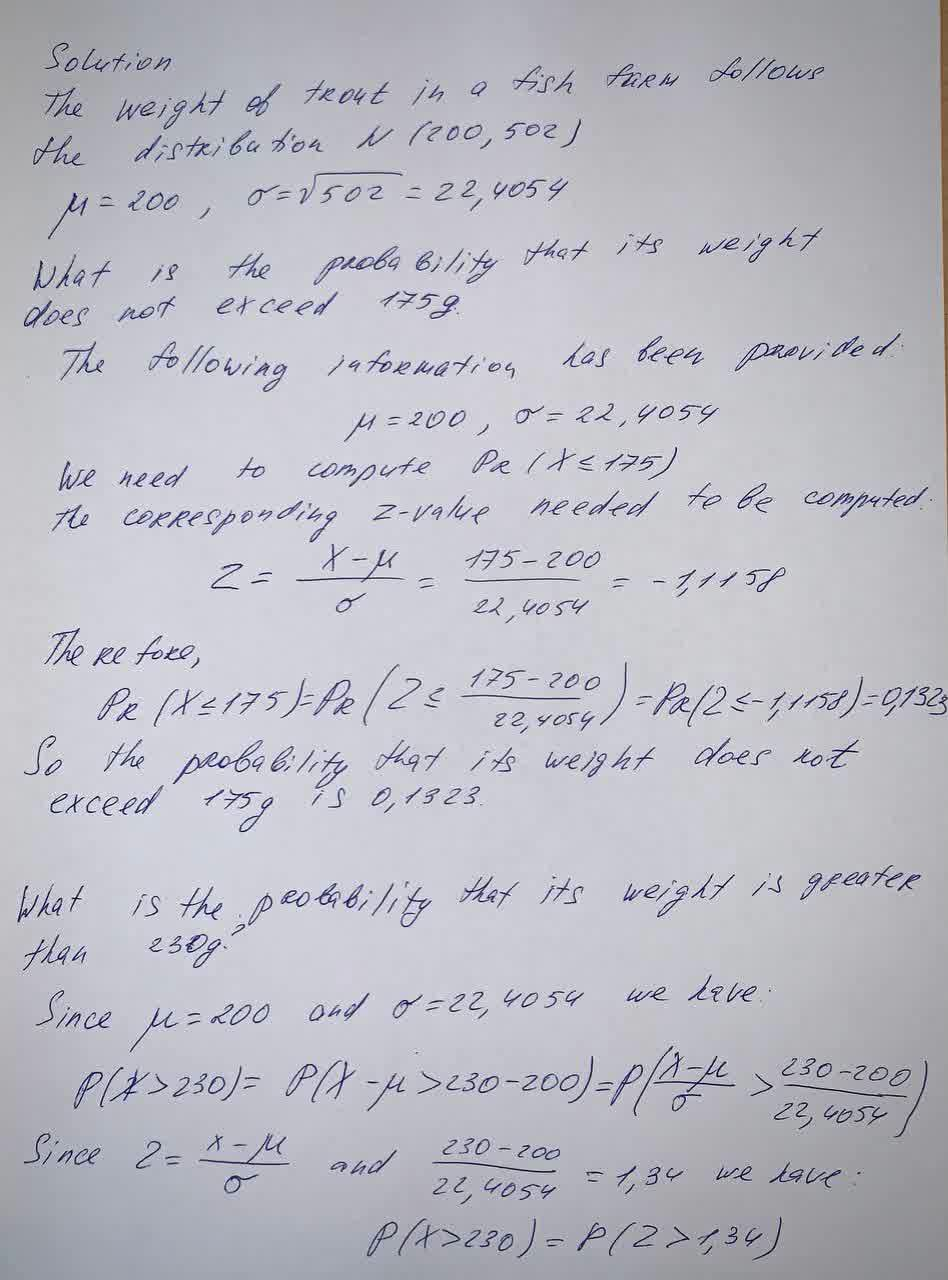What is the probability that out of eight trout selected randomly from the fish farm, less than three of them will not weigh more than 175g?Phoebe 2021-09-02 Answered
The weight of trout in a fish farm follows the distribution N (200, 502). A trout is randomly selected.
What is the probability that out of eight trout selected randomly from the fish farm, less than three of them will not weigh more than 175g?

Want to know more about Probability?

• Questions are typically answered in as fast as 30 minutes

Solve your problem for the price of one coffee

• Math expert for every subject
• Pay only if we can solve itmhalmantus
To solve: